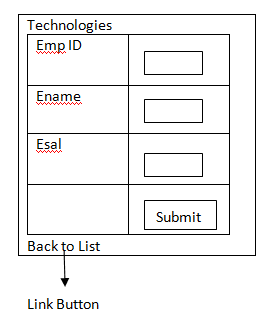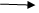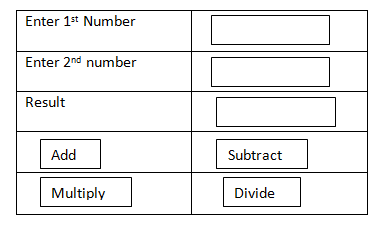# Edit records in MVC

21 September, 2018

Ratings

## Example to edit the record in MVC :-

EmpDisplay.aspx### Create  a new view . with name (EMP display aspx)Go to Source write the following code <% @ import name space = “example modes” @%> <body> <h2> technologies </h2> <div> <% HTML . Begin Forms %> <table Border = “2” align = “center”> <tr> <th> Edit  </th> <th>emp id </th><th>ename</th> <th>esal </th> <% HTML . End Form (); %> <% foreach  (var x in ()  enumerable <Emp details> model) %> <tr> <td> <% HTML. Encode  (x. emp id) %></td> <td> <% HTML. Encode  (x. ename) %></td> <td> <% HTML. Encode  (x.sal) %></td> </tr> <% } %> </table> </div> </body>Go to Home Controller. csWrite the following code for Action Method

```Public .Action Result EMP Display ()
{
Employee Entities . obj 1 = new Employee ();
Return view  (obj 1. EMP Details . To list ());
}```Go to Global .asax change action method to EMP display1.

• Great a new Strongly typed view with the name edit.aspx
• Go to source write the following code for design
```Learn ASP.net by Tekslate - Fastest growing sector in the industry.
Explore Online ASP.net Training and course is aligned with industry needs & developed by industry veterans.
Tekslate will turn you into ASP.net Expert.```

<body> <h2> technologies </h2> <div> <%  html . Begin  form (); %> <table align = “center ”  Border = “2”> <tr> <td> <label for = “emp id”> enter emp id </label>; </td> <td><% html.text box (“EMP id”) %> </td> </tr> <tr> <td> <label for = “e name”> enter ename </label>; </td> <td><% html.text box (“E name”) %> </td> </tr> <tr> <td> <label for = “e sal”> enter e sal </label>; </td> <td><% html.text box (“E salary”) %> </td> </tr> <tr> <td> <% html.Action link (Back to list , ‘Employee;’) %> </td> <td> <input = ‘submit’. Value = ‘save’> </td> </tr> </table> <% html end form (); %> </div> </body>

• Go to home controller. Cs
• Write the following code to edit action method which will get the particular EMP id record . that is transferred from EMP Display , view page, will display the data in text box.
```Public . Action Result Edit (int id)
{
Employee entities obj 1 = new employee entities ();
Var R = (from x in obj. EMP details where x. EMP id == ID Select x). first ();
Return view  (R);
}```Write the following Action method which will update the modified data update in to data base. [Accept verbs (HTTP verbs.post)]

```Public action result . edit ([bind] emp details obj edit)
{
Employee entitier. Obj 1 = new employee entitites ();
Var R =   (from x in obj1 = emp details. Where x. emp id = obj edit.emp id select x ).first ();
Obj1. Apply current values (r.entity key . entity set name, obj edit);
Obj1.save changes ();
Return. Redirect to action (“Emp display”);
}
}
}```

Example to call  the action result from multiple button  controls :Create a new mvc 2 web application  (Example 5)

## ↓

Create a new view page (action .aspx)

## ↓

Go to source write the following code <h2> sathya technologies </h2> <% HTML Begin form %> <table align = “center” border = “2”> <tr> <td> enter first number </td> <td><% HTML .text box (“T1”) %></td> </tr> <td><input type  = ‘submit’ name = ‘submit’ value = ‘Add’/> </td> <td> <input type = ‘submit’ name = ‘submit value = ‘submit’/’> </td> </tr> <tr> <td> <input type = ‘submit’ name = ‘submit value = ‘multiply/’> </td> </tr> <tr> <td> <input type = ‘submit’ name = ‘submit value = ‘divide’/’> </td> </tr> </table> <%  HTML End form () ; %> Go to Home Controller .cs à write the following code for action method.   Public Action result Arithmetic () { Return view (); }   Go to global .asax àchange the action name to arithmetic àrun the application and check Go to Home controller  write the    Arithmetic mode Following code for 4 buttons in comman. [Accept verbs  (HTTP verd.post)] Public Action result Arithmetic (string submit. String t1,  string t2) { Int a, b, c  =0; A = convert.to.int 32 (“T1”); B = convert.to.int 32 (“T2”); If (submit == “Add”) C = a +b; Else if  (submit == “submit”) C = a-b; Else if (submit == 1 multiply) C = a*b; Else if (submit ==  Divide) C = a/b; View data [‘R’] = c; Return view (); } } For indepth understanding click on